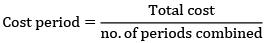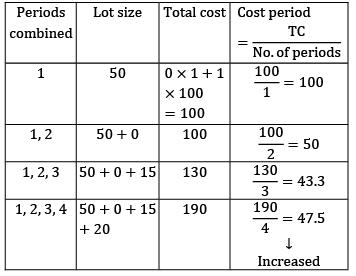Courses

# Test: APP And MRP (Level - 2)

## 10 Questions MCQ Test Manufacturing and Industrial Engineering | Test: APP And MRP (Level - 2)

Description
This mock test of Test: APP And MRP (Level - 2) for Mechanical Engineering helps you for every Mechanical Engineering entrance exam. This contains 10 Multiple Choice Questions for Mechanical Engineering Test: APP And MRP (Level - 2) (mcq) to study with solutions a complete question bank. The solved questions answers in this Test: APP And MRP (Level - 2) quiz give you a good mix of easy questions and tough questions. Mechanical Engineering students definitely take this Test: APP And MRP (Level - 2) exercise for a better result in the exam. You can find other Test: APP And MRP (Level - 2) extra questions, long questions & short questions for Mechanical Engineering on EduRev as well by searching above.
QUESTION: 1

### The master production schedule to manufacture a product is given below: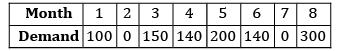If the company follows Wilson’s model to place an order. The EOQ is given as 300, the stock-on-hand is 100, lead time is 1 month. Find the minimum number of orders required to fulfill the demand arise up to month-8

Solution: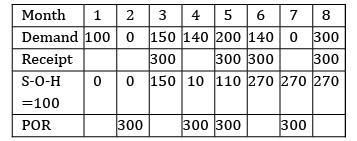Minimum number of orders required is 4

*Answer can only contain numeric values
QUESTION: 2

### The product structure of product ‘P’ is shown in the figure below. The assembly of 2 units of ‘B’ and 4 units of ‘C’ to produce 1 unit of ‘A’ takes a week.The ordering time of B, C and D are 2, 1 and 2 weeks. The master production schedule for product ‘P’ is as follows:-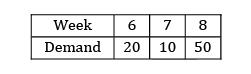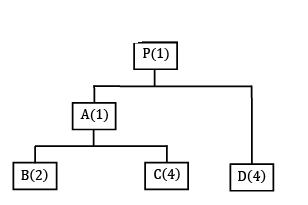The net requirement of ‘C’ if initial inventories of A, B, C and D are 10, 20, 15 and 40 is _____________

Solution:

Total demand = 80 units

For producing 1 unit of P, 80 units of ‘A’ is required but in inventory 10 units of ‘A’ is there so a total of 70 units of ‘A’ is required.

It means 4 × 70 = 280 units of C is required. But in inventory 15 units of C is there.

Therefore 280 − 15 = 265 units of ‘C’ are required.

QUESTION: 3

### Item P is made from components Q and R. Item Q, in turn is made from S and T. The lead times for items P, Q, R, S and T are 3, 4, 11, 6 and 7 weeks respectively. The lead time(in weeks) needed to respond to a customer order for item P is

Solution: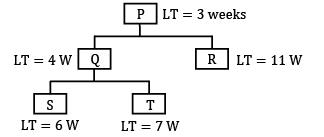P−Q−S = 6+4+3 = 13

P−R−T = 7+4+3 = 14

P−R = 11+3 = 14

QUESTION: 4

The MRP table is shown below, the POR is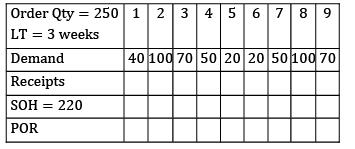Solution: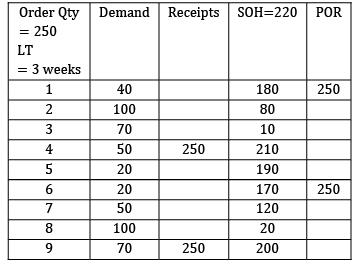QUESTION: 5

Consider the following MRP sheet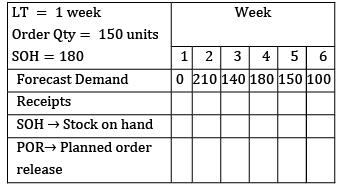The inventory at the end of 5th week

Solution: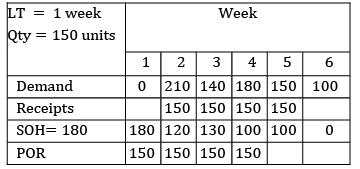Inventory at the end of 5th week = 100 units

QUESTION: 6

The material requirement planning for an item is shown in the table below: The ordering quantity is 80 units. The 2nd planned order release happens in the week of _______________

(Assume LT = 3 weeks)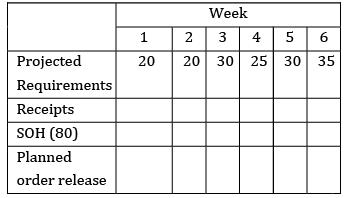Solution: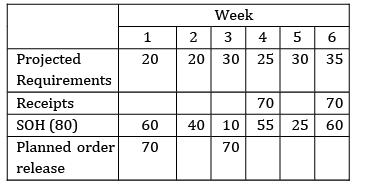QUESTION: 7

A company contains two alternative methods for manufacturing a product. The estimated time and cost for both are given below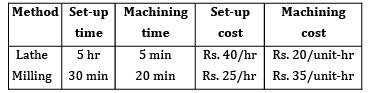The number of units up to which milling is justified economically is

Solution:

Milling operation is justified as long as the total cost is less than the lathe.

TC)Milling ≤ TC)Lathe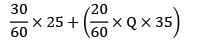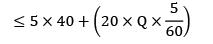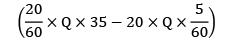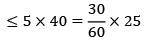10 Q ≤ 187.5

Q ≤ 18.75

Q ≤ 19

QUESTION: 8

The plant has a capacity of producing 50,000 units per year. The annual fixed cost is Rs. 80,000. The variable cost per unit is Rs.18. The price of the product is Rs. 20 per unit. Then the breakeven point in terms of the capacity of the plant is

Solution:

Given,

Fixed cost = 80,000

Variable cost = Rs. 18/unit = V

price (P) = Rs. 20/unit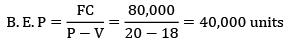Capacity of the plant = 50,000 units/year

% of BEP = 40,000/50,000 = 0.8

= 80%

QUESTION: 9

Find the total cost using Lot for Lot technique LT = 0, CO = Rs. 100/order, CC = Rs. 1/unit/week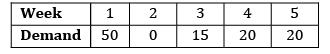Solution:

Ordering quantity = demand in a particular period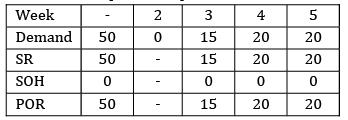TC = Stock × CC + N × CO = 0 + 4 × 100 = 400

QUESTION: 10

Find the total cost using minimum cost period technique.

LT = 0, CO = Rs. 100/order, CC = Rs. 1/unit/week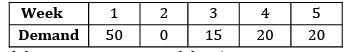Solution:

In this method cost period is used to decide the optimum combination